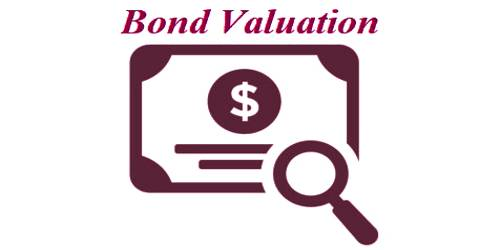# Bond Valuation

Bond valuation: It is a technique for determining the theoretical fair value of a particular bond. A bond generally provides a fixed stream of semiannual inkiest payments, plus a lump sum equal to the amount originally borrowed at maturity. As is true of all direct claim securities, a bond’s value is the present value of an expected cash flow stream, and in this case, we have the present value of an annuity plus the present value of a lump sum. Bond valuation includes calculating the present value of the bond’s cash flow (future interest payments), and the bond’s par value (value upon maturity)

The following terms are used to describe bonds.

• Par or Face Value – The par or face value of a bond is the amount of money that is paid to the bondholders at maturity.
• Coupon Rate – It is generally fixed, determines the periodic coupon or interest payments.
• Coupon Payments – It represents the periodic interest payments from the bond issuer to the bondholder.
• Maturity Date – It represents the date on which the bond matures, i.e., the date on which the face value is repaid.
• Original Maturity – The time remaining until the maturity date when the bond was issued.
• Remaining Maturity – The time currently remaining until the maturity date.
• Call Price – The amount of money the issuer has to pay to call a callable bond.
• Required Return – The rate of return that investors currently require on a bond.